# Water reservoir

The water reservoir is filled with one pump for 4 days by the second one for 9 days. Outlet can drain tank in 12 days. How long take fill the reservoir if both pumps are running and not closed outlet channel.

t =  3.6

### Step-by-step explanation:

t•(1/4+1/9-1/12)=1

10t = 36

t = 18/5 = 3.6

Our simple equation calculator calculates it.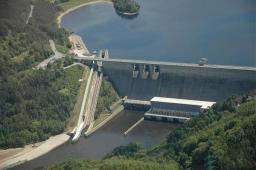Did you find an error or inaccuracy? Feel free to write us. Thank you!Tips to related online calculators
Need help to calculate sum, simplify or multiply fractions? Try our fraction calculator.
Do you have a linear equation or system of equations and looking for its solution? Or do you have a quadratic equation?
Do you want to convert time units like minutes to seconds?

## Related math problems and questions:

• Five pumpsThe water tank is filled with two pumps in 48 minutes. How long would it take to fill it with 5 same pumps?
• Three pumpsWe are filling the pool. The first pump would be filled in 12 hours, the second pump in 15 hours. If all three pumps were running at the same time, it would fill the pool for 4 hours. How long would the pool fill only with the third pump?
• Water reservoir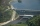One drain hole emptied the water from the tank for 20 hours, with the second drain for 30 hours. How long will the water drain from this tank if we open two drains simultaneously?
• Two pumps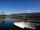The first pump fills the tank in 24 hours second pump in another 40 hours. For how long will it take to fill the tank if it operates both pumps at the same time?
• Pumps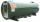6 pump fills the tank for 3 and a half days. How long will fill the tank 7 equally powerful pumps?
• Pumps A and BPump A fill the tank for 12 minutes, pump B for 24 minutes. How long will take to fill the tank if he is only three minutes works A and then both pumps A and B?
• Two pumpsPump A takes twice as long to fill a tank as B. Together they take 2 hours to fill the tank. How long does each one take to fill the tank by itself?
• PumpingThe tank is filled with a larger pump in 12 hours and with scond pump in 15 hours. For how long does the tank fill when both pumps are switched on at the same time?
• Tank 9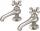The tank with volume V is filled with one pump for three hours and by the second pump for 5 hours. When both pumps run simultaneously, calculate: a) how much of the total volume of the tank is filled in one hour b) for how long is the tank full
• Tank No 8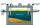Tank is filled by one inlet valve with a flow rate of 12 liters per second in 72 minutes. How long take the tank to fill, if we open half an hour after one more inlet?
• Pumps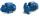Four identical pumps fill the tank in 40 hours. How many pumps would we have to use if we wanted to save 8 hours?
• The dam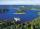The water reservoir is filled with first tributary for 25 hours second tributary for 35 hours. Some time were both inlets open, then a second tributary closed and the tank was filled in four hours. What time water flowed from both tributaries? (Expressed
• 5 pumps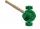Five pump to pump 3 hours 1600 hl of water. How long pumped same amount of water 4 pumps?
• Three pumps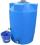The water tank is emptied with the first pump in 6 hours, with the second pump in 2 hours. In order to be emptied in 1 hour, all three pumps had to be started at once. How long will take tank be emptied by only the third pump?
• In and out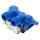The empty tank is filled in 12 minutes and empty in 16 minutes. How long does it take to fill if we forgot to close the drain? If the tank has 1000 l.
• Inlet minus outlet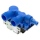Water flows into the tank through two pipes and flows out of the third pipe spontaneously. Pipe A itself would fill the tank in 3 hours, pipe B itself would fill in 4 hours, and pipe C would flow out in 12 hours. How long will the tank be full if all thre
• Five pumps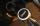Three same pumps fill the tank with 50400 liters of diesel in 7 hours. How many liters of diesel will it take in 4 hours if we add two more of the same pumps and pump them the same way? How much more (or less) will they get if we add 2 of the same pumps a